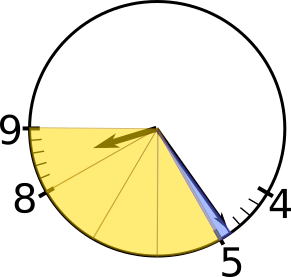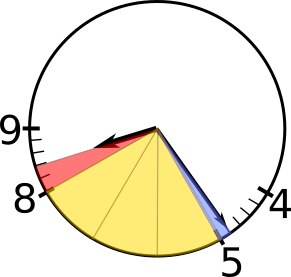#### You may also like### Hallway Borders

What are the possible dimensions of a rectangular hallway if the number of tiles around the perimeter is exactly half the total number of tiles?### Square Pegs

Which is a better fit, a square peg in a round hole or a round peg in a square hole?### Boxed In

A box has faces with areas 3, 12 and 25 square centimetres. What is the volume of the box?

# Clock Angle

##### Age 11 to 14 ShortChallenge Level
The diagram below shows the useful parts of the clock face.Working in clock minutes
We know that the minute hand has moved through 4 out of the 5 minute markers between the 4 and the 5, but as don't yet know how many of the minute markers between the 8 and the 9 the hour hand has turned through.

The hour hand will turn through 5 minute markers in 1 hour, which is 60 minutes. So it will take 60$\div$5 = 12 minutes to turn through each minute marker.

24 = 2$\times$12, so in the 24 minutes since 8 o'clock, it will have turned through 2 minute markers. This is shown on the diagram below.There are 15 minute markers between the 5 and the 8. The minute hand is one minute marker before the 5 and the hour hand is 2 minute markers after the 8, so there are 15 + 1 + 2 = 18 minute markers between the two hands.

There are 60 minute markers on the whole clock, and so 60 minute markers represent 360 degrees. 60$\times$6 = 360, so each minute marker contains 6 degrees.

So the angle between the hands is 18$\times$6$^{\text{o}}$ = 108$^{\text{o}}$.

Working in degrees
There are 60 minute markers on the clock, and so 60 minute markers represent 360 degrees. 60$\times$6 = 360, so each minute marker contains 6 degrees.

The angle between each pair of numbers shown on the clock is 6$\times$5 = 30$^{\text{o}}$, since it takes the minute hand 5 minutes to turn from one number to the next.

These angles are shown on the diagram below, where the angle in each yellow sector is 30$^{\text{o}}$ and the angle in the blue sector is 6$^{\text{o}}$.The hour hand takes 60 minutes to turn through the yellow sector between the 8 and the 9, which is a turn of 30$^{\text{o}}$. After 24 minutes, it will have turned through $\frac{24}{60}$ of the 30$^{\text{o}}$. $\frac{24}{60}=\frac{12}{30}$ so $\frac{24}{60}$ of 30$^{\text{o}}$ is the same as $\frac{12}{30}$ of 30$^{\text{o}}$, which is 12$^{\text{o}}$.

This angle is shown on the diagram below, where the angle in the red sector is 12$^{\text{o}}$ (and the angles in the yellow and blue sectors are still 30$^{\text{o}}$ and 6$^{\text{o}}$ respectively).So the angle between the minute hand and the hour hand is 6$^{\text{o}}$ + 3$\times$30$^{\text{o}}$ + 12$^{\text{o}}$, which is 108$^{\text{o}}$.
You can find more short problems, arranged by curriculum topic, in our short problems collection.